• 主要介绍了Python使用min、max函数查找二维数据矩阵中最小、最大值的方法,涉及Python矩阵生成、遍历、查找等相关操作技巧,需要的朋友可以参考下
• 适用于MAX7219 8x8 LED矩阵的MicroPython驱动程序 它是什么？ 该库支持带有MicroPython的ESP8266上的MAX7219 8x8 LED矩阵。 它在内部使用来提供图形基元和文本支持。 您可以按自己喜欢的方式链接多个矩阵：如果...
• 代码如下： a=[] for i in range(3): a.append([]) for j in range(3): ...以上这篇python for循环输入一个矩阵的实例就是小编分享给大家的全部内容了，希望能给大家一个参考，也希望大家多多支持软件开发网。
• Python实现混淆矩阵 废话少说，直接上干货： from sklearn.metrics import confusion_matrix import matplotlib.pyplot as plt plot_confusion_matrix(y_test, y_pred, classes=class_names, normalize=False) # y_...
Python实现混淆矩阵
废话少说，直接上干货：
from sklearn.utils.multiclass import unique_labels
from sklearn.metrics import confusion_matrix
import matplotlib.pyplot as plt

# y_test为真实label，y_pred为预测label，classes为类别名称，是个ndarray数组，内容为string类型的标签
class_names = np.array(["0","1"]) #按你的实际需要修改名称
plot_confusion_matrix(y_test, y_pred, classes=class_names, normalize=False)plot_confusion_matrix函数的具体实现如下，直接复制粘贴到你的代码中即可！
求各位看官老爷点个赞！
def plot_confusion_matrix(y_true, y_pred, classes,
normalize=False,
title=None,
cmap=plt.cm.Blues):
"""
This function prints and plots the confusion matrix.
Normalization can be applied by setting normalize=True.
"""
if not title:
if normalize:
title = 'Normalized confusion matrix'
else:
title = 'Confusion matrix, without normalization'

# Compute confusion matrix
cm = confusion_matrix(y_true, y_pred)
# Only use the labels that appear in the data
classes = classes[unique_labels(y_true, y_pred)]
if normalize:
cm = cm.astype('float') / cm.sum(axis=1)[:, np.newaxis]
#print("Normalized confusion matrix")
else:
pass
#print('Confusion matrix, without normalization')

#print(cm)

fig, ax = plt.subplots()
im = ax.imshow(cm, interpolation='nearest', cmap=cmap)
ax.figure.colorbar(im, ax=ax)
# We want to show all ticks...
ax.set(xticks=np.arange(cm.shape),
yticks=np.arange(cm.shape),
# ... and label them with the respective list entries
xticklabels=classes, yticklabels=classes,
title=title,
ylabel='True label',
xlabel='Predicted label')

ax.set_ylim(len(classes)-0.5, -0.5)

# Rotate the tick labels and set their alignment.
plt.setp(ax.get_xticklabels(), rotation=45, ha="right",
rotation_mode="anchor")

# Loop over data dimensions and create text annotations.
fmt = '.2f' if normalize else 'd'
thresh = cm.max() / 2.
for i in range(cm.shape):
for j in range(cm.shape):
ax.text(j, i, format(cm[i, j], fmt),
ha="center", va="center",
color="white" if cm[i, j] > thresh else "black")
fig.tight_layout()
return ax

展开全文机器学习 matplotlib
• min(0)返回该矩阵中每一列的...max(0)返回该矩阵中每一列的最大值 max(1)返回该矩阵中每一行的最大值 eg归一化算法中 minVals = dataSet.min(0) #print(minVals) #[0. 0. 0.001156] maxVals = dataSet.max(0) ...
min(0)返回该矩阵中每一列的最小值
min(1)返回该矩阵中每一行的最小值
max(0)返回该矩阵中每一列的最大值
max(1)返回该矩阵中每一行的最大值 eg归一化算法中 minVals = dataSet.min(0) #print(minVals) #[0. 0. 0.001156] maxVals = dataSet.max(0)
展开全文• 其中n*m为矩阵大小，a*b为滑动窗口大小 矩阵中的值，通过(i*j)mod 10 得到， 在滑动过程中，需要获得每次滑动窗口中的最大值，并存储下来 输出：所有最大值的和 要求及思路 纯暴力求解法，时间复杂度过高，需要...
• ## Python中矩阵运算（基于numpy包）

千次阅读 多人点赞 2019-03-11 10:15:35
博客介绍了numpy中矩阵乘法、除法运算，并补充了tensorflow中矩阵乘法运算，最后给出一个案例，分别用python和matlab实现，该案例综合运用了矩阵乘法、除法、行和、列和、向量拼接等运算
1 乘法
在数组中，a * a计算对应元素相乘（矩阵点乘）；在矩阵中，A*A计算矩阵乘法np.multiply()计算对应元素相乘（矩阵点乘）np.dot()计算矩阵乘法
import numpy as np
a=np.array([[1.,2.],[3.,4.]])
b=a*a #矩阵点乘
c=np.multiply(a,a) #矩阵点乘
d=np.dot(a,a) #矩阵乘法
print("[矩阵点乘]a*a=\n",b)
print("[矩阵点乘]multiply(a,a)=\n",c)
print("[矩阵乘法]dot(a,a)=\n",d)

A=np.mat([[1.,2.],[3.,4.]])
B=A*A #矩阵乘法
C=np.multiply(A,A) #矩阵点乘
D=np.dot(A,A) #矩阵乘法
print("\n[矩阵乘法]A*A=\n",B)
print("[矩阵点乘]multiply(A,A)=\n",C)
print("[矩阵乘法]dot(A,A)=\n",D)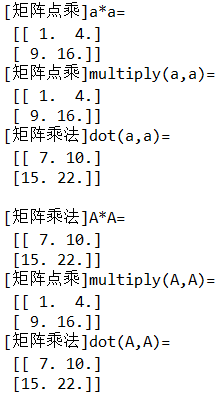补充：tensorflow中也有相应的矩阵运算
a*a计算对应元素相乘（矩阵点乘）matmul(a,a)计算矩阵乘法reduce_sum(a,0) 计算列和reduce_sum(a,1) 计算行和
import tensorflow as tf

a=tf.constant([[1.,2.],[3.,4.]])
b=a*a #矩阵点乘
c=tf.matmul(a,a) #矩阵乘法
d=tf.reduce_sum(a,0) #列和
e=tf.reduce_sum(a,1) #列和

with tf.Session() as sess:
b=sess.run(b)
c=sess.run(c)
d=sess.run(d)
e=sess.run(e)
print("[矩阵点乘]a*a=\n",b)
print("[矩阵乘法]matmul(a,a)=\n",c)
print("[列和]reduce_sum(a,0)=\n",d)
print("[行和]reduce_sum(a,1)=\n",e)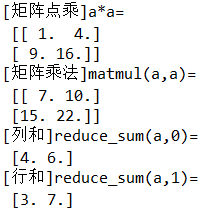2 除法
x/y计算对应元素相除（矩阵点除）np.devide()计算对应元素相除（矩阵点除）np.linalg.inv()计算矩阵的逆运算（矩阵除法）
import numpy as np
a=np.array([[1.,2.],[3.,4.]])
b=a/a #矩阵点除
c=np.divide(a,a) #矩阵点除
d=np.dot(a,np.linalg.inv(a)) #矩阵除法
print("[矩阵点除]a/a=\n",b)
print("[矩阵点除]divide(a,a)=\n",c)
print("[矩阵除法]dot(a,inv(a))=\n",d)

A=np.mat([[1.,2.],[3.,4.]])
B=A/A #矩阵点除
C=np.divide(A,A) #矩阵点除
D=np.dot(A,np.linalg.inv(A)) #矩阵除法
print("\n[矩阵点除]A/A=\n",B)
print("[矩阵点除]divide(A,A)=\n",C)
print("[矩阵除法]dot(A,inv(A))=\n",D)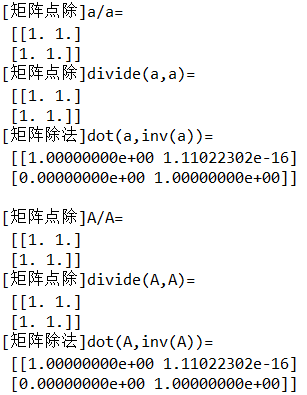3 案例
已知矩阵A，其行和、列和的最大值为max，B=(1/max)A，C=B(I-B)^(-1)，求C. Python代码：
import numpy as np

def fun(A):
m,n=np.shape(A) #获取矩阵维数
x=np.sum(A,1) #行和(列向量)
y=np.sum(A,0) #列和(行向量)
s=list(x)+list(y) #拼接数组
max_=max(s) #行和、列和中的最大值
B=A/max_
I=np.eye(m,n) #生成单位矩阵
C=np.dot(B,np.linalg.inv(I-B))
return C #函数返回值

if __name__=='__main__': #判断是否为主函数
A=np.array([[1,2],[3,4]])
C=fun(A)
print(C)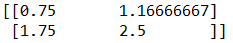另外，笔者在这里也附上Matlab代码，个人感觉还是Matlab语法更贴近自然语言。 fum.m文件（注意：Matlab要求文件名和函数名必须同名）
function C=fun(A)
[m,n]=size(A); %获取矩阵维数
for i=1:m
s1(i)=sum(A(i,:)); %计算行和
end
for j=1:n
s2(j)=sum(A(:,j)); %计算列和
end
max_=max([s1,s2]); %合并行向量、列向量，并求合并后的最大值
B=A./max_;
I=eye(m,n) %单位矩阵
C=B/(I-B);
end

demo.m文件
clear,clc %清空变量空间，并擦除命令窗口
A=[1 2;3 4];
C=fun(A)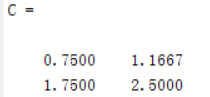展开全文• ## 灰度共生矩阵-python

万次阅读 多人点赞 2018-06-06 18:26:09
本篇博客参考灰度共生矩阵（GLCM）附Python代码 ，对其进行了完善与修改。 灰度共生矩阵（Gray Level Co-occurrence Matrix，GLCM）统计了灰度图中像素间的灰度值分布规律以区分不同的纹理。灰度共生矩阵中每个...

本篇博客参考灰度共生矩阵（GLCM）附Python代码 ，对其进行了完善与修改。

非常感谢评论区 清慎Arthur、jy2lhz、 小小菜鸡升级ing 指出代码中的问题，目前已根据建议进行修改和优化。2019-01-07

灰度共生矩阵（Gray Level Co-occurrence Matrix，GLCM）统计了灰度图中像素间的灰度值分布规律以区分不同的纹理。灰度共生矩阵中每个元素的值可以定义为(x, y)点与(x + dx, y + dy)点的值对为(i, j)的概率。统计整幅图像中每一种灰度值组合出现的概率矩阵 P 即为灰度共生矩阵。

引自百度百科 灰度共生矩阵 灰度级量化 在实际应用中，一幅灰度影像的灰度级一般为256级，在计算由灰度共生矩阵推导出的纹理特征时，要求影像的灰度级远小于256，主要是因为影像共生矩阵的计算量由影像的灰度等级和影像的大小来确定。例如:假定影像G有L个灰度级，其大小为R行C列，则运算量大约是L² * R * C，按一般情况L=256, R=512, C=512来计算，其基本运算至少要1.7 * 10十次方次。以现行微机的运算速度每秒100万次为例，对上述一幅影像计算其灰度共生矩阵至少需要1.7 X 10³秒以上，约30分钟。由此可见，这样长的时间用来进行影像的识别是不太切合实际的。解决的办法是:在尽量保持影像原形的情况下大量削减影像灰度级的取值个数和影像分辨率。因此在计算空间灰度共生矩阵时，在不影响纹理特征的前提下往往先将原影像的灰度级压缩到较小的范围，一般取8级或16级，以便减小共生矩阵的尺寸。 因为灰度级由256变为了8级，所以影像颜色显得很暗淡。但是在进行纹理信息处理时对纹理特征的影响不大。

一张图像的灰度值范围一般为0-255，为减少计算量，在计算矩阵前，需要对灰度值进行量化，一般可设置为8, 16.由于灰度共生矩阵的维度较大，一般不直接作为区分纹理的特征，而是基于它构建的一些统计量作为纹理分类特征。例如Haralick曾提出了14种基于灰度共生矩阵计算出来的统计量：能量、熵、对比度、均匀性、相关性、方差、和平均、和方差、和熵、差方差、差平均、差熵、相关信息测度以及最大相关系数。
# -*- coding: utf-8 -*-
import cv2
import numpy as np
np.set_printoptions(suppress=True)

def glcm(arr, d_x, d_y, gray_level=16):
'''计算并返回归一化后的灰度共生矩阵'''
max_gray = arr.max()
height, width = arr.shape
arr = arr.astype(np.float64)  # 将uint8类型转换为float64，以免数据失真
arr = arr * (gray_level - 1) // max_gray  # 若灰度级数大于gray_level，则将图像的灰度级缩小至gray_level，减小灰度共生矩阵的大小。量化后灰度值范围：0 ~ gray_level - 1
ret = np.zeros([gray_level, gray_level])
for j in range(height -  abs(d_y)):
for i in range(width - abs(d_x)):  # range(width - d_x)  #注释为源代码，经评论指出错误后修改
rows = arr[j][i].astype(int)
cols = arr[j + d_y][i + d_x].astype(int)
ret[rows][cols] += 1
if d_x >= d_y:
ret = ret / float(height * (width - 1))  # 归一化, 水平方向或垂直方向
else:
ret = ret / float((height - 1) * (width - 1))  # 归一化, 45度或135度方向
return ret

if __name__=='__main__':
'''归一化时分母值根据角度theta变化，0度或90度时为height * (width - 1), 45度或135度时为(height - 1) * (width - 1)'''
fp = 'C:/Users/mamq/Pictures/test photos/house_1.jpg'
img_gray = cv2.cvtColor(img, cv2.COLOR_BGR2GRAY)  # 转换为灰度图像，uint8
glcm_0 = glcm(img_gray, 1, 0)  # 水平方向
# glcm_1 = glcm(img_gray, 0, 1)  # 垂直方向
# glcm_2 = glcm(img_gray, 1, 1)  # 45度方向
# glcm_3 = glcm(img_gray, -1, 1)  # 135度方向

部分结果

[[0.0084385 0.00192513 0.00074866 0.00050802 0.00043316 0.00040107 0.00056684 0.00029947 0.00019786 0.00020856 0.00006417 0.00003209 0.00003743 0.00005348 0.00004813 0.00003209] [0.0019893 0.00362032 0.00148128 0.00079679 0.00071658 0.00060428 0.00040107 0.00027807 0.00026738 0.00017112 0.00005882 0.00006417 0.00002139 0.00003743 0.00002139 0.00005882] …… ]

以上，欢迎批评指正
展开全文纹理特征
• 灰度共生矩阵这个陌生的家伙，于是便有此文。 主要参考博客1：http://blog.csdn.net/jialeheyeshu/article/details/51337225 主要参考博客2：http://blog.csdn.net/guanyuqiu/article/details/53117507 主要参考...
• a = array([[ 1., 2., 3., 4.], [ 5., 6., 7., 8.], ...np.max(a, axis=1) #取每行的最大值 >>>array([ 4., 8., 12.]) np.max(a, axis=0) #取每列的最大值 >>>array([ 9., 10., 11., 12.])
• ## python中的矩阵运算

万次阅读 2018-09-29 11:08:48
python中的矩阵运算 摘自：http://m.blog.csdn.net/blog/taxueguilai1992/46581861 python的numpy库提供矩阵运算的功能，因此我们在需要矩阵运算的时候，需要导入numpy的包。 1.numpy的导入和使用 from numpy ....
• 没有办法在不离线更新物品相似度表的情况下将新物品退给用户 推荐理由 难以提供 可以解释 核心代码（基于ml-100k数据集） 计算相似度矩阵 def ItemCF_Norm(self): # 建立物品-物品的共现矩阵 C = dict() # 物品-物品...推荐系统 ml-100k ItemCF 协同过滤
• ## 灰度共生矩阵（GLCM）附Python代码

万次阅读 多人点赞 2018-03-06 22:47:42
灰度共生矩阵（Gray-Level Co-occurrence Matrix，GLCM）统计了灰度图中像素间的灰度值分布规律以区分不同的纹理。 度共生矩阵可以定义为一个灰度为iii的像素点与另一个与之对应位置上的像素点的灰度值为jjj的概率...OpenCV 图像纹理
• https://blog.csdn.net/mxhsyyd/article/details/80312045 min(0)返回该矩阵中每一列的最小值 min(1)返回该矩阵中每一行的最小值 max(0)返回该矩阵中每一列的最大值 max(1)返回该矩阵中每一行的最大值...
• %.^2是矩阵中的每个元素都求平方 G = G ./ max(max(G)); % Compute weights (W = G) disp('Computing weight matrices...'); %可以直接将要显示的字符放入括号中，进行表达。 % Compute Gaussian kernel (heat ...
• ## Python矩阵计算

千次阅读 2018-09-05 10:18:51
python的numpy库提供矩阵运算的功能，因此我们在需要矩阵运算的时候，需要导入numpy的包。 1.numpy的导入和使用 from numpy import *;#导入numpy的库函数import numpy as np; #这个方式使用nump...
• ## python的常见矩阵运算

万次阅读 多人点赞 2015-06-21 14:27:11
python的numpy库提供矩阵运算的功能，因此我们在需要矩阵运算的时候，需要导入numpy的包。1.numpy的导入和使用from numpy import *;#导入numpy的库函数 import numpy as np; #这个方式使用numpy的函数时，需要以np....numpy
• ## 灰度梯度共生矩阵--python

千次阅读 多人点赞 2018-06-06 18:38:34纹理特征
• np.set_printoptions(precision=2) # 在混淆矩阵中每格的概率值 ind_array = np.arange(len(classes)) x, y = np.meshgrid(ind_array, ind_array) for x_val, y_val in zip(x.flatten(), y.flatten()): c = cm[y_val...
• ## Python之max()

千次阅读 2019-04-23 14:22:45
Pythonmax() Pythonmax()函数简言之，输出为输入参数的最大值。 输入参数不能为多维矩阵，否则输出报错； >>> a array([[[ 0, 1, 2], [ 3, 4, 5]], [[ 6, 7, 8], [ 9, 10, 11]]]) >>> ...
• 矩阵用matlab代码实现maxcut：采用多种方法的Max-Cut问题解决工具 在最大割问题学者项目的背景下实现。 实施Max-Cut解决方案 maxcut.MaxCutSDP接口外部求解器（例如SCS或CVXOPT）以解决Max-Cut优化问题的SDP（半定...
• ## python计算相似矩阵

千次阅读 2018-06-08 00:10:24
模糊数学课上完之后对使用python中的sklearn计算矩阵各个元素的相似度有了一些感悟。 首先需要强调的是python中对于矩阵的计算尽量不要在DataFrame中，效率非常低。应该使用numpy。 1 预处理 常用1:...
• 内螺旋矩阵 如图所示 就是一种螺旋的形状 首先这个出现的矩阵一定是奇数来表现，然后输入你想输出的矩阵行数，必须转换为奇数 然后通过生成一个包含多个列表的列表来存储数据 生成坐标系，游标i通过判断坐标所属的...函数 数据结构
• 高斯消除矩阵 #! /usr/bin/env python # -*- coding: utf-8 -*- # def pprint(A): for i in A: print(i) print() data = [ [1, 2, 1, 2], [3, 8, 1, 12], [0, 4, 1, 2] ] n = len(data) print(输入数据) ...
• 1、矩阵的创建 由一维或二维数据创建矩阵 a1=array([1,2,3]); a1=mat(a1); data1=mat(zeros((3,3))); #创建一个3*3的零矩阵矩阵这里zeros函数的参数是一个tuple类型(3,3) data2=mat(ones((2,4))); #创建一个2*4的...
• python读取txt文件存为邻接矩阵 import numpy as np def getdata(filename): linedata = open("socfb-Amherst41.txt", 'r') #读取txt文件 cnt = 0 res = [] #存读取后的数据，二元列表 n = 2235 matrix = np....列表
• 今天学习数字图像处理课，学到灰度共生矩阵，兴起，遂敲码解之。 方法摘自百度百科： 灰度直方图是对图像上单个像素具有某个灰度进行统计的结果，而灰度共生矩阵是对图像上保持某距离的两像素分别具有某灰度的状况...数据挖掘 深度学习
• 在学习《Coding the Matrix》的时候，了解到了矩阵变换在图像中的应用，先分享coursera的视频 ...该门课程的代码在下列网站提供： ...关于矩阵变换网上有好多博文介绍，这里就只是通过python实现一下效果，也让matrix 图像处理
• 测验1：Python基本语法元素 知识点概要： 普遍认为Python语言诞生于1991年 Python语言中的缩进在程序中长度统一且强制使用，只要统一即可，不一定是4个空格（尽管这是惯例） IPO模型指：Input Process Output ......

# max矩阵pythonpython 订阅